#### 期刊菜单

Analysis of Compressive Strength and Failure Mechanism Based on Series Homogenization Model for Recycled Concrete
DOI: 10.12677/IJM.2021.102015, PDF, HTML, XML, 下载: 261  浏览: 694

Abstract: The homogenization analysis method was used to establish a series homogenization model for recycled concrete materials in the paper. A new type of finite element method—Base Force Element Method based on complementary energy principle is used to conduct meso-level damage research on recycled concrete. The stress-strain softening curve and failure mechanism of recycled concrete under uniaxial compression load are analyzed using the nonlinear damage analysis program of base force element method. The calculation results are compared with the results of experiments and meso-level random aggregate model analysis methods. The research results show that this series homogenization analysis method can be used to analyze the nonlinear damage analysis of recycled concrete materials. The compressive strength, stress-strain softening curve and crack propagation process of recycled concrete materials can be obtained using the present method.

1. 引言

2. 再生混凝土串联均质化模型

2.1. 串联均质化模型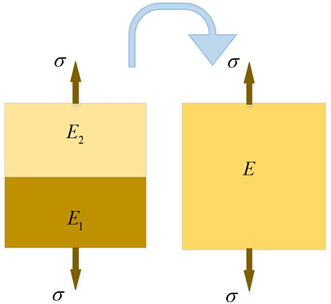Figure 1. Reuss series model

$\epsilon ={c}_{1}{\epsilon }_{1}+{c}_{2}{\epsilon }_{2}$ (1)

$\frac{\sigma }{E}={c}_{1}\frac{\sigma }{{E}_{1}}+{c}_{2}\frac{\sigma }{{E}_{2}}$ (2)

$\frac{1}{E}=\frac{{c}_{1}}{{E}_{1}}+\frac{{c}_{2}}{{E}_{2}}$ (其中 ${c}_{1}=\frac{{V}_{1}}{V}$${c}_{2}=\frac{{V}_{2}}{V}$ ) (3)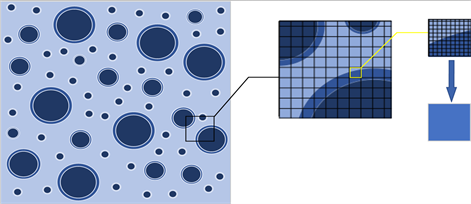Figure 2. Mesoscopic model of regenerated concrete meshes

2.2. 损伤本构模型

$\sigma ={E}_{0}\left(1-D\right)\epsilon$ (4)

$E={E}_{0}\left(1-D\right)$ (5)

$\left\{\begin{array}{l}{E}^{ag}={E}_{0}^{ag}\left(1-{D}^{ag}\right)\\ {E}^{oitz}={E}_{0}^{oitz}\left(1-{D}^{oitz}\right)\\ {E}^{m}={E}_{0}^{m}\left(1-{D}^{m}\right)\\ {E}^{om}={E}_{0}^{om}\left(1-{D}^{om}\right)\\ {E}^{\text{itz}}={E}_{0}^{itz}\left(1-{D}^{itz}\right)\end{array}$ (6)

${D}_{t}=\left\{\begin{array}{lll}0\hfill & \hfill & \text{\hspace{0.17em}}\text{\hspace{0.17em}}\text{\hspace{0.17em}}\text{\hspace{0.17em}}\text{\hspace{0.17em}}{\epsilon }_{\mathrm{max}}\le {\epsilon }_{t0}\hfill \\ 1-\frac{{\epsilon }_{t0}}{{\epsilon }_{\mathrm{max}}}+\frac{{\epsilon }_{\mathrm{max}}-{\epsilon }_{t0}}{{\eta }_{t}{\epsilon }_{t0}-{\epsilon }_{t0}}\frac{{\epsilon }_{t0}}{{\epsilon }_{\mathrm{max}}}\left(1-\mu \right)\hfill & \hfill & \text{\hspace{0.17em}}{\epsilon }_{t0}<{\epsilon }_{\mathrm{max}}\le {\eta }_{t}{\epsilon }_{t0}\hfill \\ 1-\frac{\mu }{{\xi }_{t}-{\eta }_{t}}\frac{{\epsilon }_{\mathrm{max}}-{\eta }_{t}{\epsilon }_{t0}}{{\epsilon }_{\mathrm{max}}}+\frac{\mu {\epsilon }_{t0}}{{\epsilon }_{\mathrm{max}}}\hfill & \hfill & {\eta }_{t}{\epsilon }_{t0}<{\epsilon }_{\mathrm{max}}\le {\xi }_{t}{\epsilon }_{t0}\hfill \\ 1\hfill & \hfill & \text{\hspace{0.17em}}\text{\hspace{0.17em}}\text{\hspace{0.17em}}\text{\hspace{0.17em}}\text{\hspace{0.17em}}{\epsilon }_{\mathrm{max}}>{\xi }_{t}{\epsilon }_{t0}\hfill \end{array}$ (7)

${D}_{c}=\left\{\begin{array}{lll}1-\frac{\delta }{\omega }\hfill & \hfill & \text{\hspace{0.17em}}\text{\hspace{0.17em}}\text{\hspace{0.17em}}\text{\hspace{0.17em}}{\epsilon }_{\mathrm{max}}\le \lambda {\epsilon }_{c0}\hfill \\ 1-\frac{1-\delta }{1-\lambda }\frac{{\epsilon }_{\mathrm{max}}-\lambda {\epsilon }_{c0}}{{\epsilon }_{\mathrm{max}}}-\delta \frac{{\epsilon }_{c0}}{{\epsilon }_{\mathrm{max}}}\hfill & \hfill & \text{\hspace{0.17em}}\lambda {\epsilon }_{c0}<{\epsilon }_{\mathrm{max}}\le {\epsilon }_{c0}\hfill \\ 1-\frac{1-\omega }{1-{\eta }_{c}}\frac{{\epsilon }_{\mathrm{max}}-{\epsilon }_{c0}}{{\epsilon }_{\mathrm{max}}}-\frac{{\epsilon }_{c0}}{{\epsilon }_{\mathrm{max}}}\hfill & \hfill & \text{ }\text{ }{\epsilon }_{c0}<{\epsilon }_{\mathrm{max}}\le {\eta }_{c}{\epsilon }_{c0}\hfill \\ 1-\frac{\omega {\epsilon }_{c0}}{{\epsilon }_{\mathrm{max}}}\hfill & \hfill & {\eta }_{c}{\epsilon }_{c0}<{\epsilon }_{\mathrm{max}}\le {\xi }_{c}{\epsilon }_{c0}\hfill \\ 1\hfill & \hfill & \text{\hspace{0.17em}}\text{\hspace{0.17em}}\text{\hspace{0.17em}}\text{\hspace{0.17em}}{\epsilon }_{\mathrm{max}}>{\xi }_{c}{\epsilon }_{c0}\hfill \end{array}$ (8)

$\epsilon =\frac{\sigma }{{E}_{m}}={c}_{0}\frac{\sigma }{{E}_{0}}+{c}_{1}\frac{\sigma }{{E}_{1}}+{c}_{2}\frac{\sigma }{{E}_{2}}+{c}_{3}\frac{\sigma }{{E}_{3}}+{c}_{4}\frac{\sigma }{{E}_{4}}=\sigma \left(\frac{{c}_{0}}{{E}_{0}}+\frac{{c}_{1}}{{E}_{1}}+\frac{{c}_{2}}{{E}_{2}}+\frac{{c}_{3}}{{E}_{3}}+\frac{{c}_{4}}{{E}_{4}}\right)$ (9)

$\frac{1}{{E}_{eq}}=\frac{{c}_{0}}{{E}_{0}^{m}\left(1-{D}^{m}\right)}+\frac{{c}_{1}}{{E}_{0}^{ag}\left(1-{D}^{ag}\right)}+\frac{{c}_{2}}{{E}_{0}^{oitz}\left(1-{D}^{oitz}\right)}+\frac{{c}_{3}}{{E}_{0}^{om}\left(1-{D}^{om}\right)}+\frac{{c}_{4}}{{E}_{0}^{itz}\left(1-{D}^{itz}\right)}$ (10)

$\frac{1}{{E}^{eq}}=\left\{\begin{array}{l}\frac{{c}_{0}}{{E}_{0}^{m}}+\frac{{c}_{1}}{{E}_{0}^{ag}}+\frac{{c}_{2}}{{E}_{0}^{em}}\text{}\epsilon \le {\epsilon }_{0}^{em}\\ \frac{{c}_{0}}{{E}_{0}^{m}}+\frac{{c}_{1}}{{E}_{0}^{ag}}+\frac{{c}_{2}}{{E}_{r}^{em}}\text{}{\epsilon }_{0}^{em}<\epsilon \le {\epsilon }_{0}^{m}\\ \frac{{c}_{0}}{{E}_{r}^{m}}+\frac{{c}_{1}}{{E}_{0}^{ag}}+\frac{{c}_{2}}{{E}_{r}^{em}}\text{}{\epsilon }_{0}^{m}<\epsilon \le {\epsilon }_{0}^{ag}\\ \frac{{c}_{0}}{{E}_{r}^{m}}+\frac{{c}_{1}}{{E}_{r}^{ag}}+\frac{{c}_{2}}{{E}_{r}^{em}}\text{}{\epsilon }_{0}^{ag}<\epsilon \le {\epsilon }_{r}^{em}\\ \frac{{c}_{0}}{{E}_{r}^{m}}+\frac{{c}_{1}}{{E}_{r}^{ag}}+\frac{{c}_{2}}{{E}_{u}^{em}}\text{}{\epsilon }_{r}^{em}<\epsilon \le {\epsilon }_{r}^{m}\\ \frac{{c}_{0}}{{E}_{u}^{m}}+\frac{{c}_{1}}{{E}_{r}^{ag}}+\frac{{c}_{2}}{{E}_{u}^{em}}\text{}{\epsilon }_{r}^{m}<\epsilon \le {\epsilon }_{r}^{ag}\\ \frac{{c}_{0}}{{E}_{u}^{m}}+\frac{{c}_{1}}{{E}_{u}^{ag}}+\frac{{c}_{2}}{{E}_{u}^{em}}\text{}{\epsilon }_{r}^{ag}<\epsilon \le {\epsilon }_{u}^{em}\\ \frac{{c}_{0}}{{E}_{r}^{m}}+\frac{{c}_{1}}{{E}_{r}^{ag}}\text{}{\epsilon }_{u}^{em}<\epsilon \le {\epsilon }_{u}^{m}\\ \frac{{c}_{1}}{{E}_{r}^{ag}}\text{}{\epsilon }_{u}^{m}<\epsilon \le {\epsilon }_{u}^{ag}\\ 0\text{}{\epsilon }_{u}^{ag}<\epsilon \end{array}$ (11)Table 1. Material parameter value

3. 再生混凝土立方体单轴静态压缩数值模拟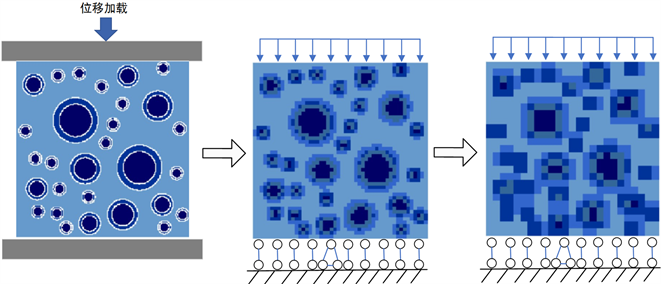Figure 3. Load modelFigure 4. Two-dimensional diagram of homogenized modelTable 2. Numerical simulation results data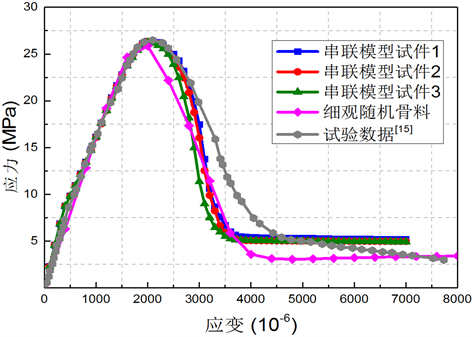Figure 5. Uniaxial compression stress-strain curve of series model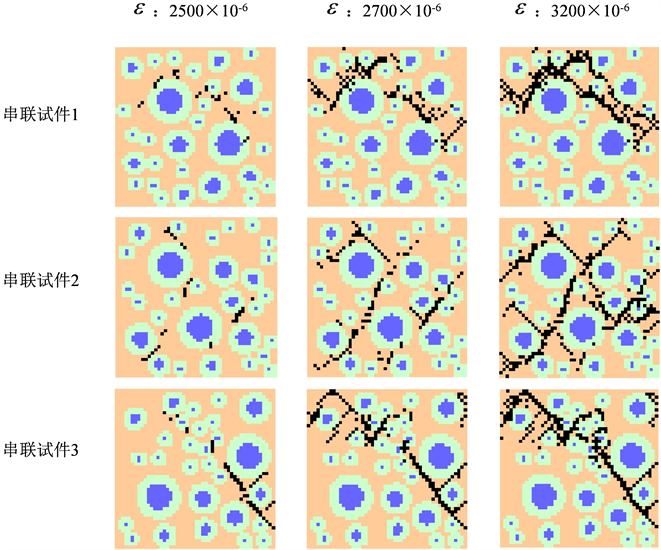Figure 6. Uniaxial compression numerical simulation damage diagram

4. 结论

1) 利用均质化分析方法，推导出均质化模型的串联等效本构关系，建立了再生混凝土材料的多折线损伤本构模型。

2) 基于余能原理基面力元法，开发了再生混凝土均质化分析的非线性基面力元分析软件和均质化前处理软件。

3) 采用串联等效的均质化模型对再生混凝土的单轴压缩试验进行了数值计算分析，得到了应力–应变软化曲线和损伤破坏过程。

4) 通过将本方法的结果与试验数据的对比，验证了模型建立的可行性与合理性。

5) 研究表明，采用均质化模型的计算效率大大提高，远远高于基于随机骨料模型的细观损伤分析方法，且能保证一定的计算精度。

  肖建庄. 再生混凝土创新研究与进展[M]. 北京: 科学出版社, 2020.  肖建庄, 杜江涛, 刘琼. 基于格构模型再生混凝土单轴受压数值模拟[J]. 建筑材料学报, 2009, 12(5): 511-514, 518.  李文贵, 肖建庄, 袁俊强. 模型再生混凝土单轴受压应力分布特征[J]. 同济大学学报(自然科学版), 2012, 40(6): 906-913.  Peng, Y. and Liu, Y. (2019) Advances in the Base Force Element Method. Springer, Singapore. https://doi.org/10.1007/978-981-13-5776-3  彭一江, 应黎坪. 再生混凝土细观分析方法[M]. 北京: 科学出版社, 2018.  Liu, C., Xing, L., Liu, H., Nong, X. and Xu, X. (2021) Experimental on Repair Performance and Complete Stress-Strain Curve of Self-Healing Recycled Concrete under Uniaxial Loading. Construction and Building Materials, 285, Article ID: 122900. https://doi.org/10.1016/j.conbuildmat.2021.122900  Choubey, R.K., Kumar, S. and Chakradhara Rao, M. (2016) Modeling of Fracture Parameters for Crack Propagation in Recycled Ag-gregate Concrete. Construction and Building Materials, 106, 168-178. https://doi.org/10.1016/j.conbuildmat.2015.12.101  谢永滨, 平乐, 李荣彬, 杨虹, 吴苗苗, 李丹丹, 罗冬梅. 再生混凝土单轴受压应力-应变全曲线分析[J]. 混凝土与水泥制品, 2018(2): 95-100.  Anuruddha, J., Adams, M.P. and Bandelt, M.J. (2018) Understanding Variability in Recycled Aggregate Concrete Mechanical Properties through Numerical Simulation and Statistical Evaluation. Construction and Building Materials, 178, 301-312. https://doi.org/10.1016/j.conbuildmat.2018.05.158  Thomas, J., Nazeer Thaickavil, N. and Wilson, P.M. (2018) Strength and Durability of Concrete Containing Recycled Concrete Aggregates. Journal of Building Engineering, 19, 349-365. https://doi.org/10.1016/j.jobe.2018.05.007  Tan, X., Li, W., Zhao, M. and Tam, V.W.Y. (2019) Numerical Discrete-Element Method Investigation on Failure Process of Recycled Aggregate Concrete. Journal of Materials in Civil Engineering, 31, Article ID: 04018353. https://doi.org/10.1061/(ASCE)MT.1943-5533.0002562  Guo, M., Grondin, F. and Loukili, A. (2019) Nu-merical Method to Model the Creep of Recycled Aggregate Concrete by Considering the Old Attached Mortar. Cement and Concrete Research, 118, 14-24. https://doi.org/10.1016/j.cemconres.2019.01.008  Kazemian, F., Rooholamini, H. and Hassani, A. (2019) Mechanical and Fracture Properties of Concrete Containing Treated and Untreated Recycled Concrete Aggregates. Construction and Building Materials, 209, 690-700. https://doi.org/10.1016/j.conbuildmat.2019.03.179  Alireza, M., Rahimzadeh, O.P. and Arul, A. (2021) Dis-crete Element Modeling of Cemented Recycled Concrete Aggregates under Unconfined and k0 Loading Conditions. Transportation Geotechnics, 26, Article ID: 100450. https://doi.org/10.1016/j.trgeo.2020.100450  肖建庄. 再生混凝土单轴受压应力-应变全曲线试验研究[J]. 同济大学学报(自然科学版), 2007, 35(11): 1445-1449.